# Periodic Solutions to the Lorenz Equations

Changing the value of a parameter in the equations that produce the famous Lorenz chaotic attractor yields nonlinear ordinary differential equations that have periodic solutions.

### Contents

#### The Lorenz equations

(This section is adapted from chapter 7 of my book Numerical Computing with MATLAB, published by MathWorks and SIAM.) The Lorenz chaotic attractor was first described in 1963 by Edward Lorenz, an M.I.T. mathematician and meteorologist who was interested in fluid flow models of the earth's atmosphere. An excellent reference is the book by Colin Sparrow cited at the end of this blog. I have chosen to express the Lorenz equations in a somewhat unusual way involving a matrix-vector product: $$\dot{y} = A y$$ Here $y$ is a vector-valued function of $t$ with three components and $A$ is a 3-by-3 matrix that depends on $y$. Seven of the nine elements in $A$ are constant, but the other two depend on $y_2(t)$: $$A = [\ -\beta \ \ 0 \ \ y_2 \ ; \ \ 0 \ \ -\sigma \ \ \sigma \ ; \ \ -y_2 \ \ \rho \ \ -1 \ ]$$ The first component of the solution, $y_1(t)$, is related to the convection in the atmospheric flow, while the other two components are related to horizontal and vertical temperature variation. The parameter $\sigma$ is the Prandtl number, $\rho$ is the normalized Rayleigh number, and $\beta$ depends on the geometry of the domain. The most popular values of the parameters are $\sigma = 10$, $\beta = 8/3$, and $\rho = 28$. These are outside the ranges associated with the earth's atmosphere. The deceptively simple nonlinearity introduced by the presence of $y_2$ in the system matrix $A$ changes everything. There are no random aspects to these equations, so the solutions $y(t)$ are completely determined by the parameters and the initial conditions, but their behavior is very difficult to predict. For some values of the parameters, the orbit of $y(t)$ in three-dimensional space is known as a strange attractor. It is bounded, but not periodic and not convergent. It never intersects itself. It ranges chaotically back and forth around two different points, or attractors. For other values of the parameters, the solution might converge to a fixed point, diverge to infinity, or oscillate periodically. Think of $\eta = y_2$ as a free parameter, restrict $\rho$ to be greater than one, and study the matrix $$A = [\ -\beta \ \ 0 \ \ \eta \ ; \ \ 0 \ \ -\sigma \ \ \sigma \ ; \ \ \eta \ \ \rho \ \ -1 \ ]$$ It turns out that $A$ is singular if and only if $$\eta = \pm \sqrt{\beta (\rho-1)}$$ The corresponding null vector, normalized so that its second component is equal to $\eta$, is $$[\ \rho-1 \ \ \eta \ \ \eta \ ]^T$$ With the two different signs for $\eta$, this defines two points in three-dimensional space. These points are fixed points for the differential equation. If either of these points is taken as the initial condition, then the initial derivative $\dot{y}(0) = 0$ and so $y(t)$ never changes. Fix $\sigma = 10$, $\beta = 8/3$, and investigate the effect of the parameter $\rho$. For $\rho$ less than about 24.7, the fixed points are stable. If the initial value is close enough, the orbit will spiral in to the fixed point. However, for larger values of $\rho$ these points are unstable. If $y(t)$ does not start at one of these points, it will never reach either of them. For most values of $\rho$ greater than 24.7, including the popular $\rho = 28$, the orbit is chaotic.

#### Periodic solutions

In his comprehensive study of the Lorenz equations, Colin Sparrow found four exceptional values of $\rho$ where a stable periodic orbit arises out of the chaos. If the initial point happens to be anywhere on the orbit, the solution will return to that point in finite time. If the initial point is not on the orbit, the trajectory will converge towards the periodic orbit. This behavior is unusual for nonlinear equations. Each orbit has what might be called a characteristic signature. Each of the four orbits has a different signature. Use "+" and "-" to denote the sign of $\eta$ in the null vector. In the three-dimensional plots below, the red dots are these attractors. The point with the "+" is on the upper left, while the "-" is on the lower right.

#### Rho = 99.65

This is the most complex orbit. Its signature is +--+--. It circles the "+" fixed point once and then the "-" fixed point twice. Then it circles the "+" fixed point once again and the "-" point twice again on a slightly different trajectory before repeating the orbit.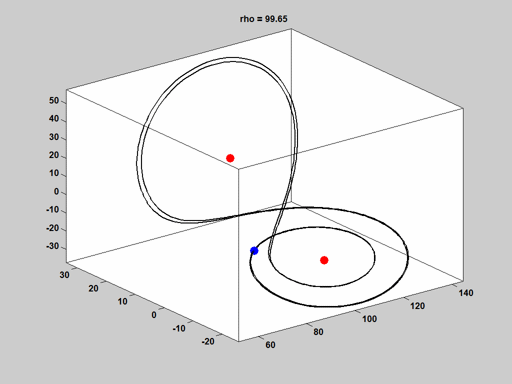#### Rho = 100.5

The signature is ++-. Circle "+" twice, then "-" once.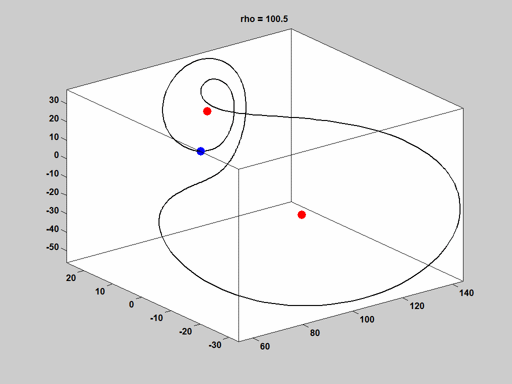#### Rho = 160

The signature is ++--. Circle each fixed point twice before heading towards the other.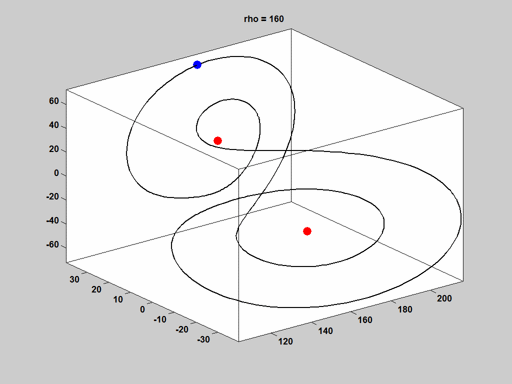#### Rho = 350

The simplest orbit. The signature is +-. No fuss, no bother.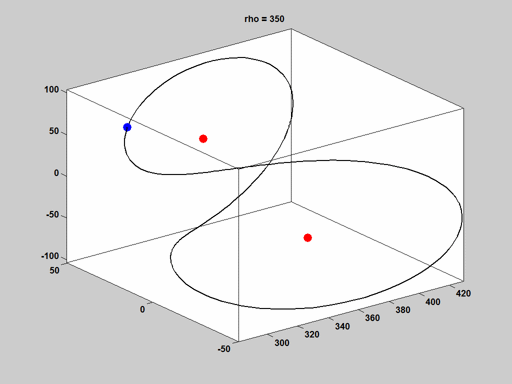#### Rho = 28

In case you haven't seen it before, here is a plot for the value of $\rho$ that is usually studied, $\rho = 28$. This is the well known Lorenz butterfly. To really appreciate it, you should literally get hold of a three-dimensional plot and view it from different angles. This trajectory goes on forever, never intersecting itself, and never getting too close to, or too far away from, the two unstable fixed points. Most values of $\rho$ near $\rho = 28$ produce similar chaotic behavior.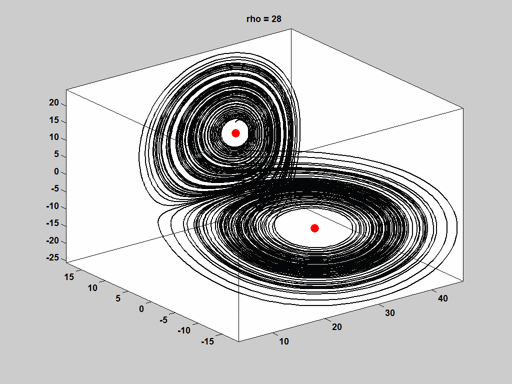#### lorenzgui

If you want to see these orbits in action, in 3-D, download and run lorenzgui.m.

#### References

Colin Sparrow, The Lorenz Equations: Bifurcations, Chaos, and Strange Attractors, Springer, 1982, <http://link.springer.com/book/10.1007%2F978-1-4612-5767-7> James Gleick on Chaos: Making a New Science, YouTube interview, https://www.youtube.com/watch?v=3orIIcKD8p4 James Gleick, Chaos: Making a New Scince, Penguin Books, 1987, <http://www.penguin.com/book/chaos-by-james-gleick/9780143113454>,

Published with MATLAB® R2014a

|

### コメント

コメントを残すには、ここ をクリックして MathWorks アカウントにサインインするか新しい MathWorks アカウントを作成します。